## 密钥消除攻击

Schnorr密钥聚合一文中，是最简单的聚合方式，现在可以进一步说下了。 这种方式的前提是要求参与者都是诚实的，实际实现中要加入额外的公钥验证，否则会出现安全问题。 下面说下可能的安全问题： 假设有两个参与者A和B，$P_A,PB$分别是二者的公钥。 假设B不诚实，参与密钥聚合过程中，提供假的公钥$P{FB}=P_B-P_A$, 导致聚合公钥： $P=PA+P{FB}=P_A+P_B-P_A=P_B$ ，

1. 参与者公钥hash聚合 $L = H(P_A+P_B）$
2. 生成聚合公钥 $P =H(L,P_A)P_A+H(L,P_B)P_B$
3. 生成聚合随机数 $R =R_A+R_B$
4. 生成聚合签名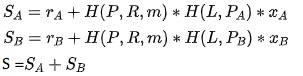5. (R, S)即最后得到的签名，验证如下： S G = R + H(P, R, m) P

## BLS m of n门限签名

BLS 使用了不同方法实现门限签名，以 2-3 多重签名为例说明（可扩展为任意的 m-n 多重签名）。

### 准备阶段：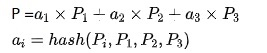$MK_i=(a_1 x_1) q_i + (a_2 x_2) q_i+ (a_3 x_3) q_i$

$e(G,MK_i)=e(P,q_i)$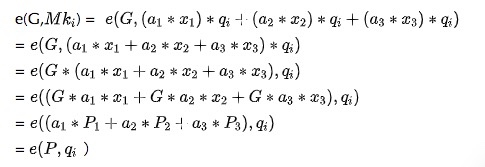### 签名阶段：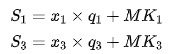### 验证阶段：

$e(G, S’) =e(P',H(p,m)) * e(P,q_1 + q_3)$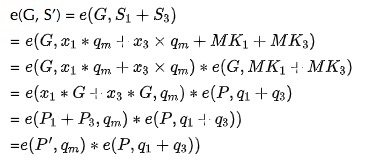## 小结

### 相关阅读：

Schorr签名与椭圆曲线 Schorr签名与椭圆曲线

• 发表于 2020-12-26 23:22
• 阅读 ( 2746 )
• 学分 ( 5 )
• 分类：入门/理论